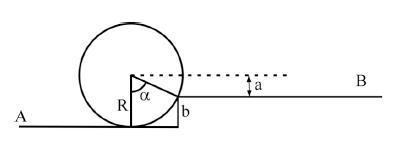# Mechanics: Cylinder rolling up a step without slipping

## Homework Statement

A uniform cylinder of radius R, length L and density $$\delta$$ is rolling without slipping along a horizontal surface with constant centre of mass speed u at A. It then meets a step of height b. We wish to find the conditions under which the cylinder is able to continue past the step and roll along the surface B.Assuming no energy is lost due to friction or drag, the change in the total kinetic energy before and after must be equal and opposite to the change in gravitational potential energy. Find a requirement on the initial speed u such that the cylinder passes B with positive kinetic energy.

## Homework Equations

As the disc is rolling without slipping it is possible to use $$v = \omegaR$$.

The total kinetic energy is given by

$$\frac{1}{2}I\omega^2 + \frac{1}{2}mx^2$$

where

$$I = \frac{1}{2}MR^2$$

and gravitational potential is given by $$mgh$$.

## The Attempt at a Solution

You are told in the question that $$\Delta K = -\Delta P$$

The change in kinetic energy will be negative and thus the change in potential will be positive. I want the final kinetic energy to be positive thus

$$K > |\Delta K|$$.

That's about as far as I have gotten. Any tips on how to get started will be greatly appreciated.

Also, I'm not sure if $$\alpha$$ is significant or not (the $$\alpha$$ on the diagram, not the angular acceleration).

NB: Sorry about my equations, I'm still trying to get used to using LaTeX.

Last edited:

Hootenanny
Staff Emeritus
Gold Member
Welcome to PF iopmar06,

Thanks for taking the time to properly layout your question.

The total kinetic energy is given by

$$\frac{1}{2}I\omega^2 + \frac{1}{2}mx^2$$

where

$$I = \frac{1}{2}MR^2$$
Good. Now using these equations can you explicitly write an expression for the total kinetic energy of the cylinder before the step, using the information given in the question?

Can you also write an expression for the change in potential energy of the cylinder, again using the variables you are given in the question?

I think I may have worked it out.

Kinetic Energy is given by:

$$K = \frac{1}{2} I \omega^2 + \frac{1}{2} m v^2 = \frac{1}{2} (\frac{1}{2} m R^2) \omega^2 + \frac{1}{2} mv^2$$

Then:

$$\Delta P = mg \Delta h = mga$$

$$\Delta K = - \Delta P = -mga$$

We want the kinetic energy of the cylinder rolling along B to be positive so

$$\frac{1}{4} mr^2 \omega^2 + \frac{1}{2}mv^2 -mga > 0$$

$$\frac{1}{2} mv^2 > mga - \frac{1}{4} mr^2 \omega^2$$

$$v^2 > 2ga - \frac{(r \omega)^2}{2}$$

Last edited:
So then the condition on the initial velocity is:

$$v > \sqrt{2ga - \frac{(r \omega)^2}{2}}$$

Hootenanny
Staff Emeritus
Gold Member
I think I may have worked it out.

Kinetic Energy is given by:

$$K = \frac{1}{2} I \omega^2 + \frac{1}{2} m v^2 = \frac{1}{2} (\frac{1}{2} m R^2) \omega^2 + \frac{1}{2} mv^2$$

Then:

$$\Delta P = mg \Delta h = mga$$

$$\Delta K = - \Delta P = -mga$$

We want the kinetic energy of the cylinder rolling along B to be positive so

$$\frac{1}{4} mr^2 \omega^2 + \frac{1}{2}mv^2 -mga > 0$$

$$\frac{1}{2} mv^2 > mga - \frac{1}{4} mr^2 \omega^2$$

$$v^2 > 2ga - \frac{(r \omega)^2}{2}$$
Looks good so far. However, you are not given $\omega$ explicitly. Therefore, you need to express the angular velocity in terms of the quantities that you are given (HINT: There are still a few pieces of information you haven't used, but you only need one of them).

Last edited:
That completely slipped my mind, thanks for pointing it out.

The cylinder is not slipping so

$$v = \omega r$$

Substituting this into the inequality gives

$$v^2 > 2ga - \frac{1}{2} (r \frac{v}{r})^2$$

$$v^2 > \frac{4ga}{3}$$

so

$$v > 2 \sqrt{ \frac{ga}{3}}$$

Hootenanny
Staff Emeritus
Gold Member
That completely slipped my mind, thanks for pointing it out.

The cylinder is not slipping so

$$v = \omega r$$

Substituting this into the inequality gives

$$v^2 > 2ga - \frac{1}{2} (r \frac{v}{r})^2$$

$$v^2 > \frac{4ga}{3}$$

so

$$v > 2 \sqrt{ \frac{ga}{3}}$$
Not a problem. Looks good to me. However, as a minor point don't forget that you are asked to find a condition for u and not v.

Last edited:
Yeah I'm just in the habit of using v for velocity.

Anyway, thanks a lot for all your help.

Hootenanny
Staff Emeritus